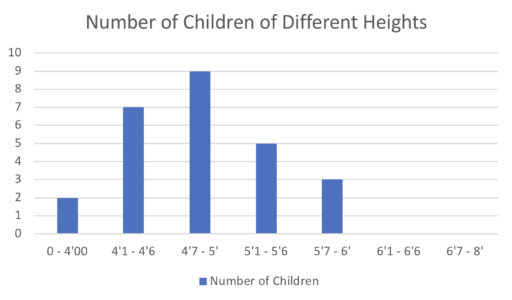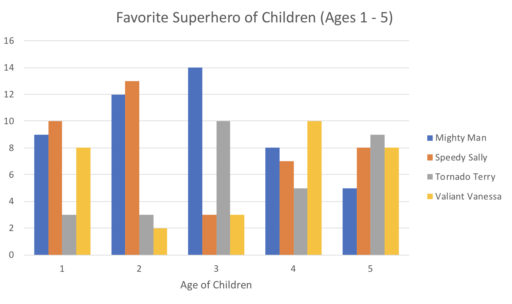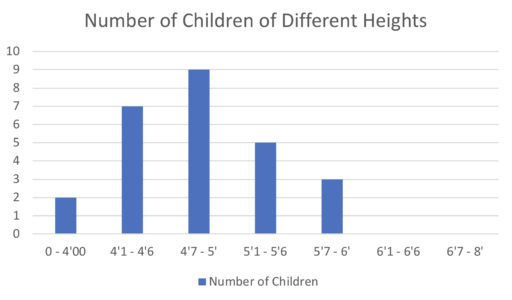A bar graph tells you how many objects in a given category you have. For example, in the following bar graph:Each bar tells you how many children fall into that height range. So, for example, there are 2 children between 0 and 4 feet; there are 7 between 4 feet 1 inch and 4 feet 6 inches, and so on.

Example 1

How many children are between 4 feet 7 inches and 6 feet? How many children are taller than 6 feet?

We can also have segmented bar graphs like the following:These bar graphs allow us to compare different groups, in this case the group of 1 year olds against the group of 2 year olds, 3 year olds, and so on. We can thus see how their preferences shift over time.

To read the graph, the horizontal signs tell us which categories we have (here, the different ages 1 to 5) and then the vertical component tell us how many members of that category (e.g. how many one year-olds) in accordance with the key to the right. Thus, the left-most blue bar tells us how many 1 year-olds have "Mighty Man" as their favorite superhero. The right-most yellow bar tells us how many 5 year-olds have "Valiant Vanessa" as their favorite hero and so on.

Example 2

Which superhero gains the most fans as the children mature from being 1 years-old to 5 years-old?

Example 3

Which superhero has the most supporters overall (i.e. across all the age groups)?

Example 4Which of the following tables goes with the given bar graph:

A.B.C.D.Practice Problems

1. Which of the following tables is compatible with the given graph?A.B.C.D.2. A new kid, Richard, joins the class. Richard is 4'8. Given that the graph below accurately depicts the heights of Richard's classmates, what is the maximum possible number of classmates that are taller than Richard? What is the minimum possible number?3. In the below chart, what percentage of three-year olds take Tornado Terry to be their favorite hero?4. In the below chart, which superhero loses the most fans as children age from 1 to 5?5. In the below chart, what if anything can you conclude about the median height of the class?